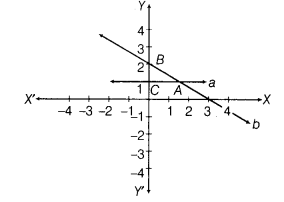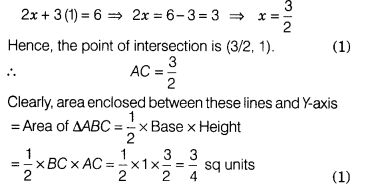# A student wrote the equations of the lines a and h drawn in the following graph

A student wrote the equations of the lines a and h drawn in the following graph as y = l and 2x + 3y = 6, respectively. Is he right? If yes, then write the coordinates of point of intersection of lines a and b.Also, find the area enclosed between these lines and F-axis.

Clearly, line a is parallel to X-axis at a distance of 1 unit in positive direction of Y-axis, therefore its equation is y = 1.
Also, if we draw the graph of line 2x + 3y = 6, then its graph should intersect X-axis at (3, 0) and Y-axis at (0, 2) and so the given equation of line b is correct.
Thus, the student is right.
Now, substituting y = 1 in equation of line b, we get